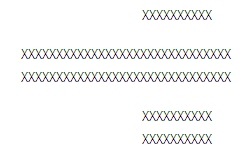﻿ Variance Property (LineRemoveCommand) | Leadtools.ImageProcessing.Core | Raster, Medical, Document Help
←Select platform
In This Topic ▼

# Variance Property

Summary
Gets or sets the amount of width change that is tolerated between adjacent line slices.
Syntax
C#
Objective-C
C++/CLI
Java
Python
``public int Variance { get; set; } ``
``@property (nonatomic, assign) NSInteger variance; ``
````public int getVariance(); `
`public void setVariance( `
`   int intValue `
`); ````
````public: `
`property int Variance { `
`   int get(); `
`   void set (    int ); `
`} ````
``Variance # get and set (LineRemoveCommand) ``

#### Property Value

Amount of width change that is tolerated between adjacent line slices. If LineRemoveCommandFlags.UseDpi is set in the Flags property, units are in thousandths of an inch, otherwise units are in pixels. This property is valid only if LineRemoveCommandFlags.UseVariance is set in the Flags property. For example, the following horizontal line is 30 pixels in length (X represents a pixel)If Variance is 2 pixels (i.e. tolerate width changes of 2 pixels or less), the first 10 columns and the last 10 columns of the line will be removed. The middle 10 columns will be unchanged. This helps preserve characters that intersect a line that is being removed.
Example
C#
````using Leadtools; `
`using Leadtools.Codecs; `
`using Leadtools.ImageProcessing.Core; `
` `
`public void ImageRegionPropertyExample() `
`{ `
`   // Load an image `
`   RasterCodecs codecs = new RasterCodecs(); `
`   codecs.ThrowExceptionsOnInvalidImages = true; `
` `
`   RasterImage image = codecs.Load(Path.Combine(LEAD_VARS.ImagesDir, "Clean.tif")); `
` `
`   // Prepare the command `
`   LineRemoveCommand command = new LineRemoveCommand(); `
`   command.LineRemove += new EventHandler<LineRemoveCommandEventArgs>(LineRemoveEvent_S3); `
`   command.Type = LineRemoveCommandType.Horizontal; `
`   command.Flags = LineRemoveCommandFlags.UseVariance | LineRemoveCommandFlags.SingleRegion; `
`   command.GapLength = 2; `
`   command.MaximumLineWidth = 5; `
`   command.MinimumLineLength = 200; `
`   command.MaximumWallPercent = 10; `
`   command.Wall = 7; `
`   command.Variance = 2; `
`   command.Run(image); `
` `
`} `
` `
`private void LineRemoveEvent_S3(object sender, LineRemoveCommandEventArgs e) `
`{ `
`   MessageBox.Show("Row Col " + "( " + e.StartRow.ToString() + ", " + e.StartColumn + " )" + `
`      "\n Length " + e.Length.ToString()); `
`   e.Status = RemoveStatus.Remove; `
`} `
` `
`static class LEAD_VARS `
`{ `
`   public const string ImagesDir = @"C:\LEADTOOLS22\Resources\Images"; `
`} ````
Requirements